# Deriving the Cubic Formula Detailed Steps Included

Knoji reviews products and up-and-coming brands we think you'll love. In certain cases, we may receive a commission from brands mentioned in our guides. Learn more.
This article derives the solution to the cubic equations. It provides all the missing steps that most articles gloss over and never provide the details.

A general cubic equation can be represented as Equation #1 below. The only restrictions on the coefficients are as follows:

A) All coefficients must be real.

B) The leading coefficient associated with the cubic must be non-zero in value; otherwise, there is no cubic equation!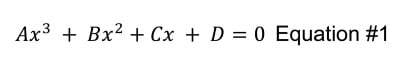Step #1: The first step is to eliminate the squared term. This is called DEPRESSING THE CUBIC.

Depressing the cubic is accomplished by making the following substitution and consolidating terms. The substitution is listed in Equation 2 below. This method was first introduced by Nicolo Fontana Tartaglia (1500 to 1557).The next step is to expand, combine and eliminate terms.

Note: All terms will be listed from left to right in decreasing order of the variable. All terms will be written with coefficients to the left of the variables. The coefficients will be alphabetically increasing from left to right; that is: the term “BA” will be written as “AB.”

The quickest method is to expand the first and second terms in Equation #3. The problem is that the computations will be somewhat messy. This can be simplified a little by making a quick substitution as listed in Equation #4 and then substituting the final terms back into Equation #3.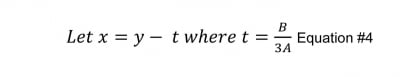At this point we can expand the generic terms and then substitute the value for “t.” Recall after using the binomial expansion we get the following: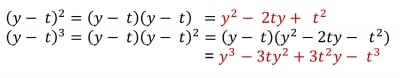Now substituting the terms used in Equation #4 we have: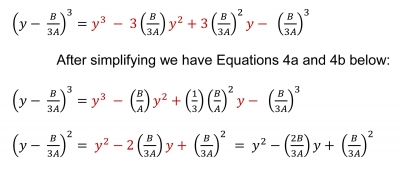Now substituting Equations 4a and 4b back into #3 and simplifying. For simplicity each group is solved seperately.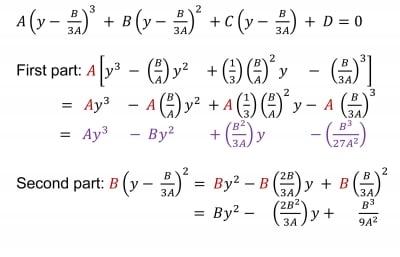The last term can be re-written whereas the denominator includes 27 in it. Recall if you multiply the fraction by 1 you do not change the value of the fraction. In this case, we need to multiply by the equivalent of one or 3/3. Thus: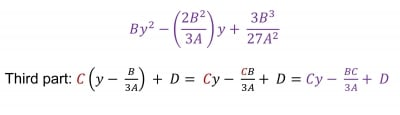Combing all three parts and aligning them vertically we have: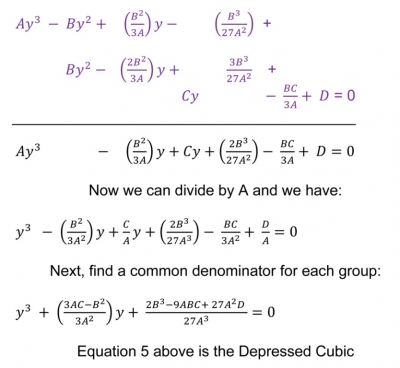For simplicity sake we can momentarily simplify Equation 5 with the subsequent substitutions: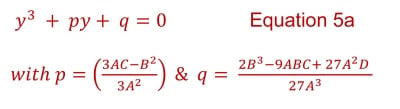Step #2: The Ferro substitution discovered by Scipione del Ferro (1465 to 1526).

In this substitution we want to find s & t such that the following is true: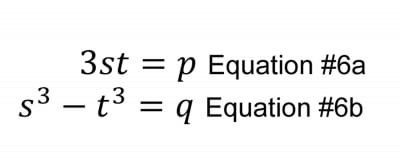When this is done we have a solution to Equation 5a in the form y = t – s. A simple check will show this is the solution after all the substitutions in Equations 6a, 6b and y = t – s are plugged into Equation 5a.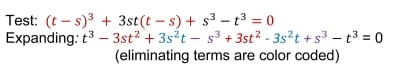Step #2B: We first solve for t in Equation #6a and plug the results into Equation #6b: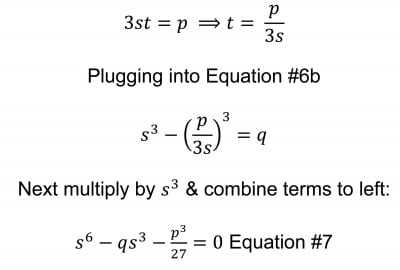Letting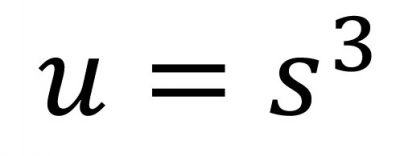we can reduce Equation #7 into a simple quadratic and use the quadratic formula to solve it. Thus: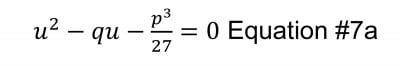Now, we plug in the values of “q” and “p” into Equations #7a and generate Equation #8 below: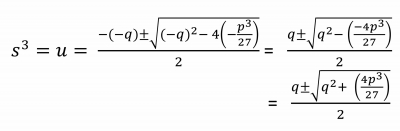At this point we want to solve the quadratic in terms of s & t. We do this by plugging the values for p & q found in equations 6a and 6b into Equation #8.We have the following: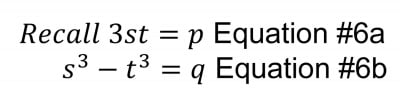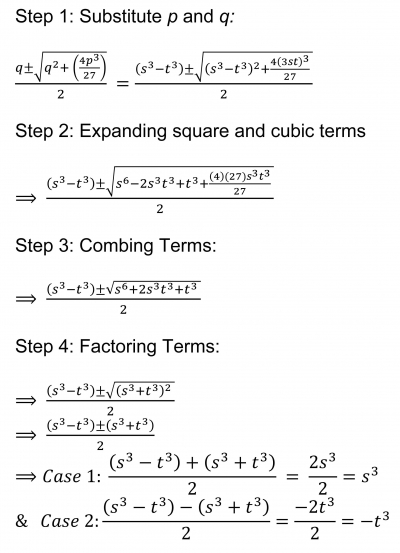Therefore we have found two independent roots to Equation 8: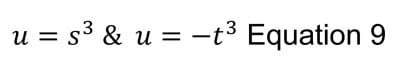Solving for s & t in Equation 9 we have: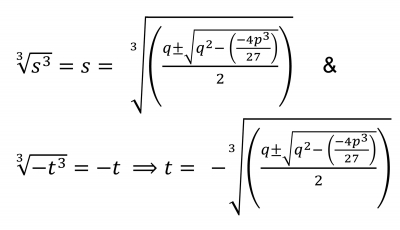Equations 9a and 9b listed above.

Step #3: Taking Equations 9a and 9b and back substituting to the original variables we have: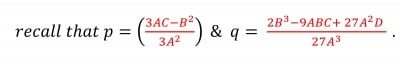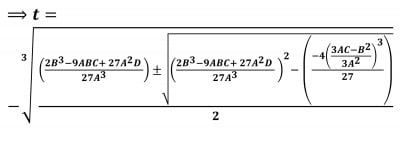The above Equation is labeled 10a

First step is to clearly show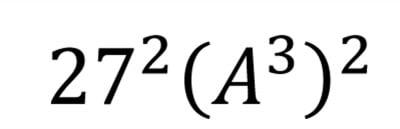is in the denominator for the inside quadratic formula. Recall

that: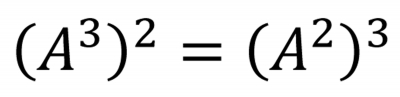Since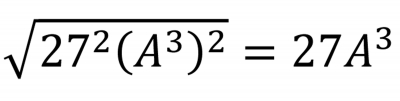then the term can then be pulled out of the quadratic formula as follows: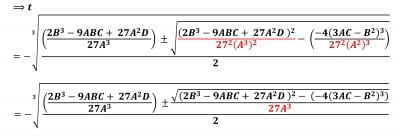The next two steps are: pull out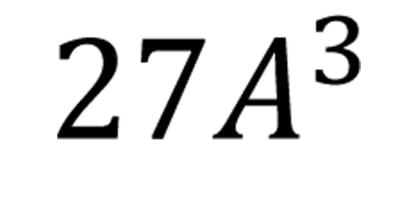from the denominator of the cubic root and to distribute the negative in the second term of the square root. Recall: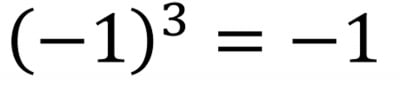. The result is: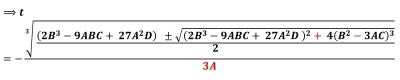The above Equation is labeled 10

Step 4: The final solution.

Since y = t - s is a solution to the equation we have Equation 11 below: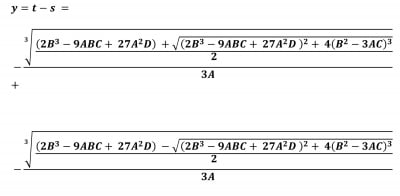Above equation is labeled #11

Equation #11 is the solution to the Depressed Cubic, not for the original equation #1. The final solution is made when we recall that: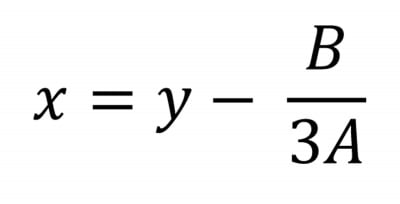Therefore all that is needed is to subtract the B/3A. From Equation #11 and we have the real value solution to equation. Thus: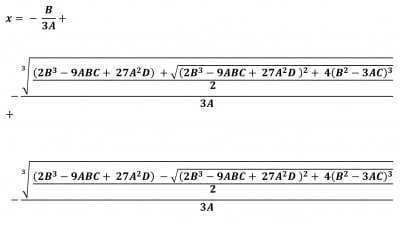The above equation is labeled #12.

With Equation #12 we are able to find one root of the cubic equation. The other two roots can be found by using synthetic division into the original cubic equation. The resulting polynomial will be of degree two and the two roots can be easily determined using the quadratic formula.

One needs to note that the cubic equation has limitations when the operand is negative. However the following wiki article http://en.wikipedia.org/wiki/Cubic_formula can better describe other methods and techniques that can be used.

Hopefully this mathematical presentation provides a useful outline when you need to derive the cubic formula.

Take Care!

Other math and science articles by the same author:

Deriving the Quadratic Formula by Completing the Square Method

How to Add Formulas Tables and other pdf Files into your Factoidz Articles

How to Read and use Roman Numerals

Estimating the Definite Integral using Riemann Sums

The Basics on how Stellites Orbit the Earth